# Segmenting data with segmentr

#### 2019-01-09

Segmentr is a package that implements a handful of algorithms to find segments that maximize its total likelihood. So, the user of this package has to find an adequate likelihood for the segmentation problem to be solved, possibly having to penalize it as well in order to avoid a solution biased towards either too big or too small segments. Also, it’s important to consider the computation time of each algorithm and its tradeoffs. This vignette walks through the main concepts regarding its usage, using weather temperature from Berlin as an example. For this demo, the following packages will be used:

require(segmentr)
require(tidyr)
require(tibble)
require(dplyr)
require(lubridate)
require(magrittr)

## Understanding the data

The berlin dataset, provided in this dataset, contains daily temperature data from several weather stations in Berlin.

data(berlin)
berlin[, 1:5]
#>                    2010-01-01 2010-01-02 2010-01-03 2010-01-04 2010-01-05
#> Berlin-Buch              -1.6       -1.9       -4.8       -6.2       -6.1
#> Berlin-Dahlem (FU)       -1.9       -2.3       -4.5       -6.2       -6.8
#> Berlin-Kaniswall         -1.9       -2.0       -4.6       -6.6       -7.7
#> Berlin-Marzahn           -1.8       -1.9       -4.8       -6.2       -6.2
#> Berlin-Schoenefeld       -1.9       -2.1       -4.6       -6.5       -7.0
#> Berlin-Tegel             -1.5       -2.0       -4.5       -6.0       -5.5
#> Berlin-Tempelhof         -1.4       -1.7       -4.3       -5.6       -6.0

In order to grasp how the data behave, look at the how the daily mean temperature behaves over time.

berlin %>%
colMeans() %>%
enframe("time", "temperature") %>%
mutate_at(vars(time), ymd) %>%
with(plot(time, temperature, type="l"))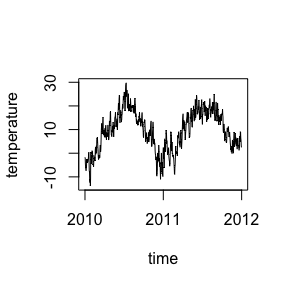It’s possible to mean weather cycles every year. A “linear regression likelihood” function will be used to segment the this data and find when the trends change.

## Building the likelihood

To build an adequate likelihood function that uses a linear regression, it’s necessary to find a metric that is higher the better fit a segment is. Turns out the adjusted r-squared is a metric used to measure how well a linear model fits the data, so it will be used.

A segmentr likelihood function takes a segment as a matrix and returns the likelihood of the segment, so it’s necessary to build a function with that interface.

lm_likelihood <- function (data) {
as_tibble(t(data)) %>%
rowid_to_column() %>%
gather(station, temperature, -rowid) %>%
with(lm(temperature ~ rowid)) %>%
summary %>%
.$adj.r.squared } c(lm_likelihood(berlin[, 2:3]), lm_likelihood(berlin[, 1:150]), lm_likelihood(berlin)) #>  0.98211599 0.76014938 0.03283039 The lm_likelihood function returns a low value when compared to a hypothetical slice of the first 150 columns, so it appears reasonable. However, the highest likelihood was obtained with a segment of size 2, which indicates this function has a tendency to favor small data. f <- Vectorize(. %>% floor() %>% { berlin[, 1:.] } %>% lm_likelihood) curve(f, from = 1, to = 730)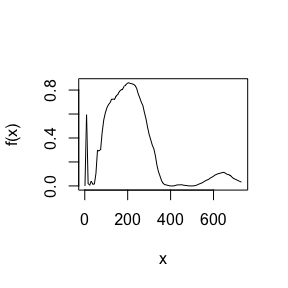This graph represents how the likelihood of the first $$x$$ segments in the data, and one can see the curve has a peak close to zero. Even though it’s not the global maximum of the curve, the cumulative effect of all the segments in the data is enough to bias the solution towards small segments. ## Penalizing the likelihood function To penalize small segments, it’s important to penalize the likelihood function to avoid non-wanted behavior. Segmentr provides the auto_penalize utility, which returns a penalized version of the likelihood function. penalized_likelihood <- auto_penalize(berlin, lm_likelihood) f <- Vectorize(. %>% floor() %>% { berlin[, 1:.] } %>% penalized_likelihood) curve(f, from = 1, to = 730)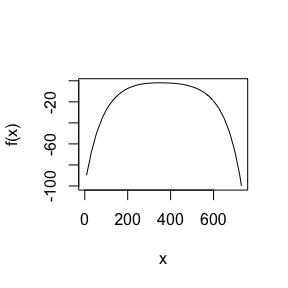The new likelihood curve penalizes segments too big or too small, indicating it might work as we expect. ## Segmenting the data With the penalized likelihood at hand, it’s finally possible to segment the data. For that, the “hierarchical” algorithm will be used, which manages to segment the data in a reasonable $$O(n \log(n))$$ time complexity, by assuming the data to have a hierarchical structure. It returns reasonable results most of the times, but there is always the risk it doesn’t split the data correctly. To find the exact answer, the “exact” algorithm would have to be used, but it’s quite costly with $$O(n^2)$$. results <- segment( berlin, likelihood = penalized_likelihood, algorithm = "hierarchical" ) results #> Segments (total of 2): #> #> 1:351 #> 352:730 It looks the segments are still rather large, so the penalty on small segments might be too large. The penalty function can be tuned to adjust it. penalized_likelihood <- auto_penalize(berlin, lm_likelihood, big_segment_penalty = 10, small_segment_penalty = 1.1) results <- segment( berlin, likelihood = penalized_likelihood, algorithm = "hierarchical" ) results #> Segments (total of 4): #> #> 1:189 #> 190:328 #> 329:551 #> 552:730 Plot the actual change points on the daily means to have a better understanding of how the segments split the data. dates <- colnames(berlin) %>% ymd() berlin %>% colMeans() %>% enframe("time", "temperature") %>% mutate_at(vars(time), ymd) %>% with({ plot(time, temperature, type="l") abline(v=dates[results$changepoints], col="red", lty=2)
})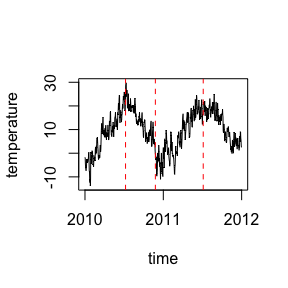They more or less split the data as expected.

## Limitations of the “hierarchical” algorithm

Even though it runs fast, the “hierarchical” algorithm should be handled with care, because it assumes the data has a hierarchical segment structure, which is not always true. To give an example, a subset of the berlin dataset is used.

sub_berlin <- berlin[, 1:551]

results <- segment(
sub_berlin,
likelihood = penalized_likelihood,
algorithm = "hierarchical"
)

results
#> Segments (total of 2):
#>
#> 1:281
#> 282:551

The “hierarchical” algorithm only splits the data in half in this situations. This happens because it first tries to find a optimal change point in the data, so that it can recursively find the remainder.

sub_berlin %>%
colMeans() %>%
enframe("time", "temperature") %>%
mutate_at(vars(time), ymd) %>%
with({
plot(time, temperature, type="l")
abline(v=dates[results\$changepoints], col="red", lty=2)
})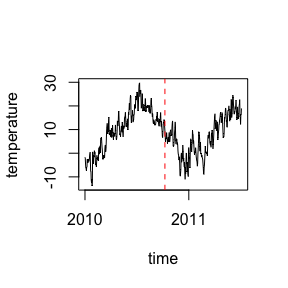The problem with that is that it thinks the data is optimally split roughly at the middle of the dataset, when in fact it would require putting change points in roughly each one third of the data. Hence, the hierarchical algorithm is not able to optimally split the dataset.